# Relationships

Materials for class on Tuesday, September 26, 2017

## Miscellaneous R stuff

Download Comic Relief data and place it in a folder named `data` in your project: Comic Relief 2015–2016 grants

### Searching for code on GitHub

GitHub is an excellent source of real-world code, and it’s easy to search for examples of things other people have written. Use the advanced search to limit the search language to R.

### Installing packages from GitHub

Not all R packages are hosted on CRAN—many are repositories that live on GitHub. It’s possible to install packages from GitHub, but you can’t use RStudio’s “Packages” panel. Most non-CRAN packages will include instructions of how to install (such as this package for ridge plots), but the general syntax is this:

``````library(devtools)

# Or, in one line

# So, in real life, you can do this:
devtools::install_github("clauswilke/ggridges")
devtools::install_github("andrewheiss/reconPlots")``````

### Pipes (`%>%`)

Pipes let you concatenate nested functions in a human-readable form and can make it a lot easier to understand your code:

``````library(tidyverse)

# Create a bunch of numbers
x <- rnorm(1000)

# Nest some functions
round(mean(x), digits = 2)
sqrt(var(x))
round(sqrt(var(x)), digits = 2)

# Use pipes to make nested functions easier to read and write
x %>%
var() %>%
sqrt() %>%
round(digits = 2)``````

Pipes are even more helpful when manipulating data frames. For instance, typically it’s best to load the raw data as a data frame, clean it with a chain of functions, and then save it as a separate data frame:

``````library(tidyverse)
library(lubridate)

cr <- cr_raw %>%
rename(amount = `Amount Awarded`,
duration = `Planned Dates:Duration (months)`,
program = `Grant Programme:Title`,
award_date = `Award Date`) %>%
# year() comes from lubridate and it extracts the year from a date object
mutate(award_year = year(award_date)) %>%
# as.factor() converts values to categorical variables
mutate(award_year = as.factor(award_year))``````

### Working with ggplot objects

You can save the output of a `ggplot` plot to an object (`p1` here):

``````# Get rid of the largest grants
cr_filtered <- cr %>%
filter(amount < 3000000)

p1 <- ggplot(cr_filtered, aes(x = duration, y = amount)) +
geom_point(aes(color = award_year)) +
scale_color_manual(values = c("green", "brown")) +
geom_smooth(method = "loess", color = "#274981") +
geom_smooth(method = "lm")
p1``````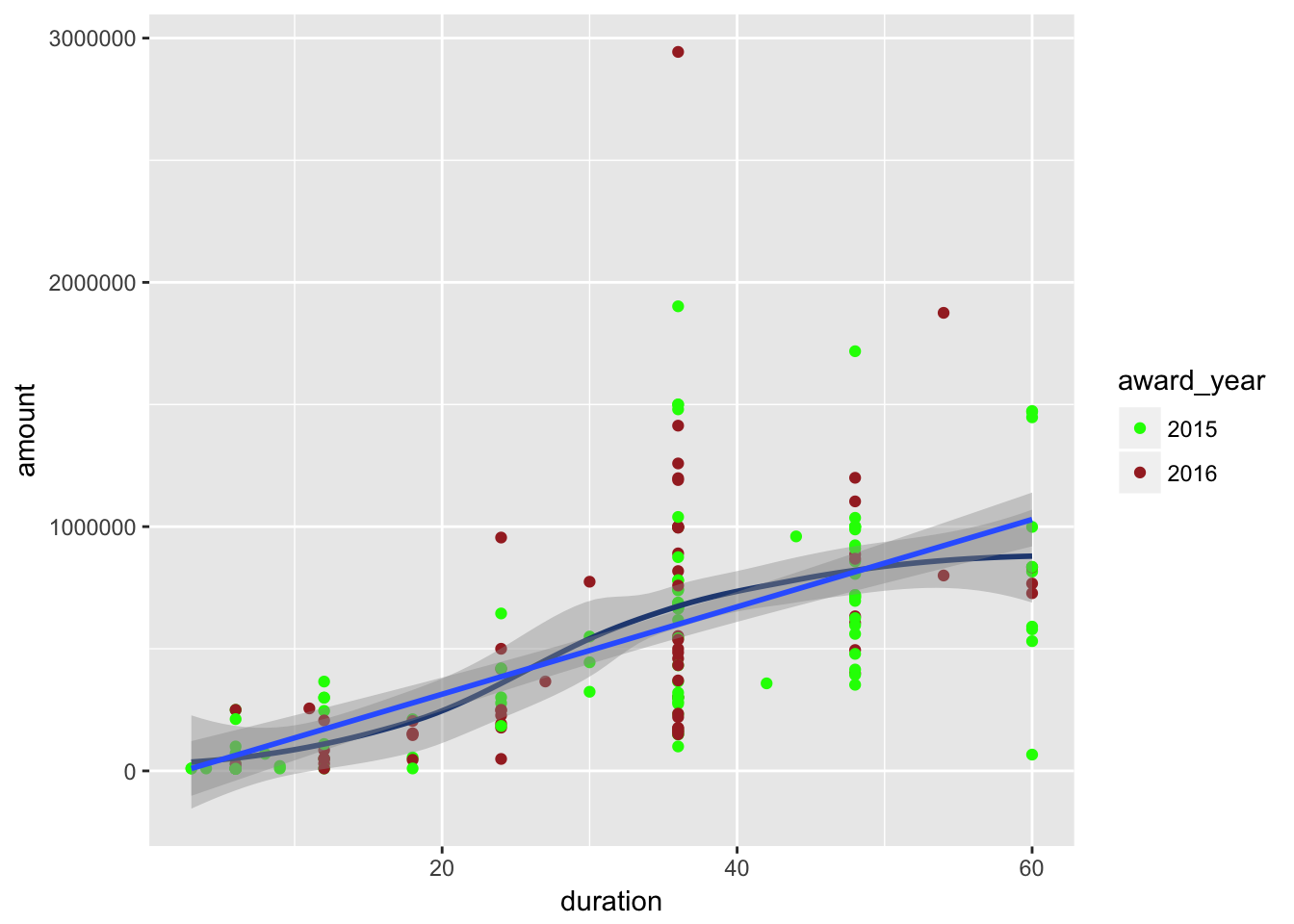When you save `ggplot` plots as objects, you can do fun things with them, like add additional layers without repeating all the code from the base plot:

``````p2 <- p1 +
labs(title = "Some cool title")
p2``````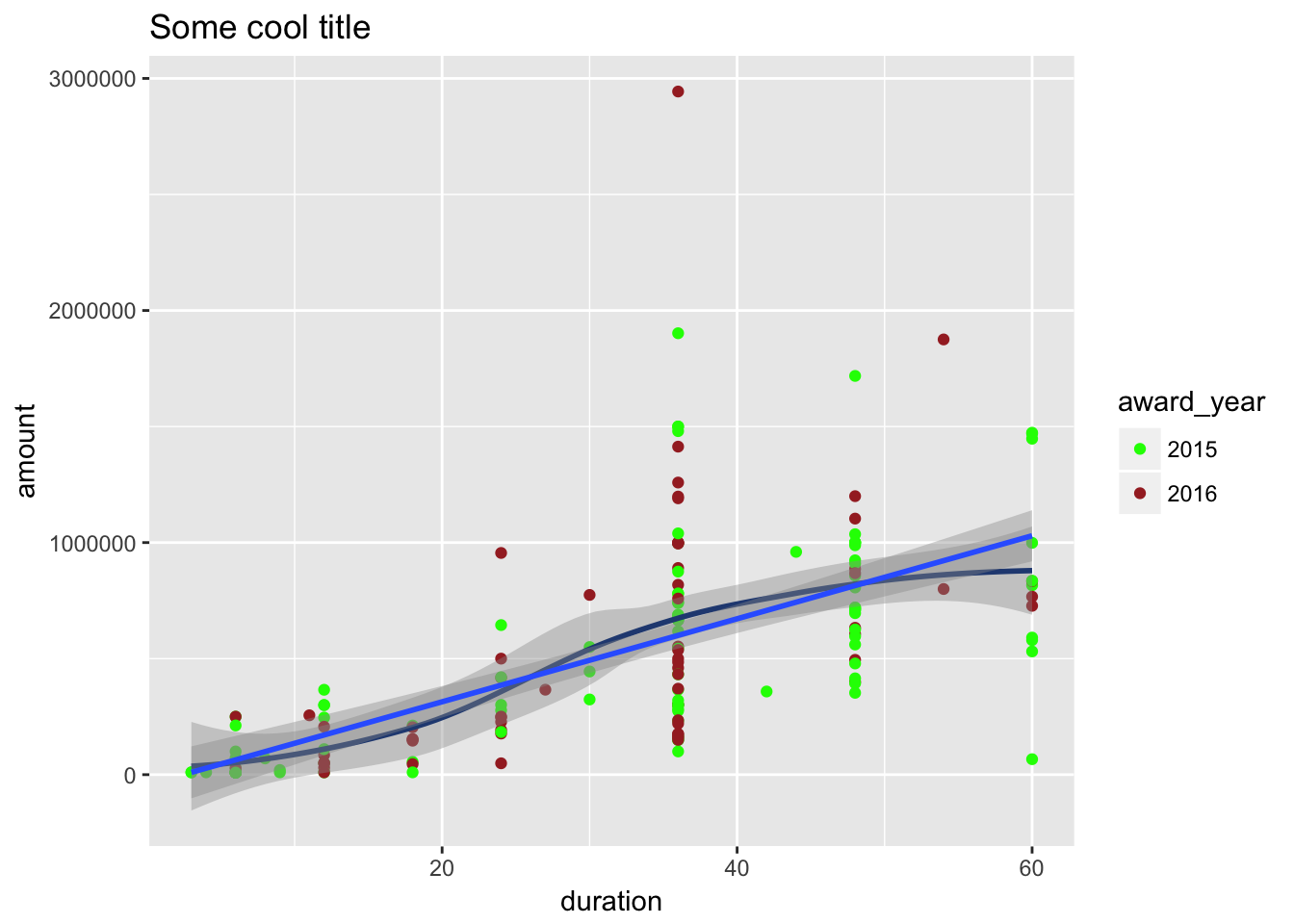``````p3 <- p2 +
theme_bw()
p3``````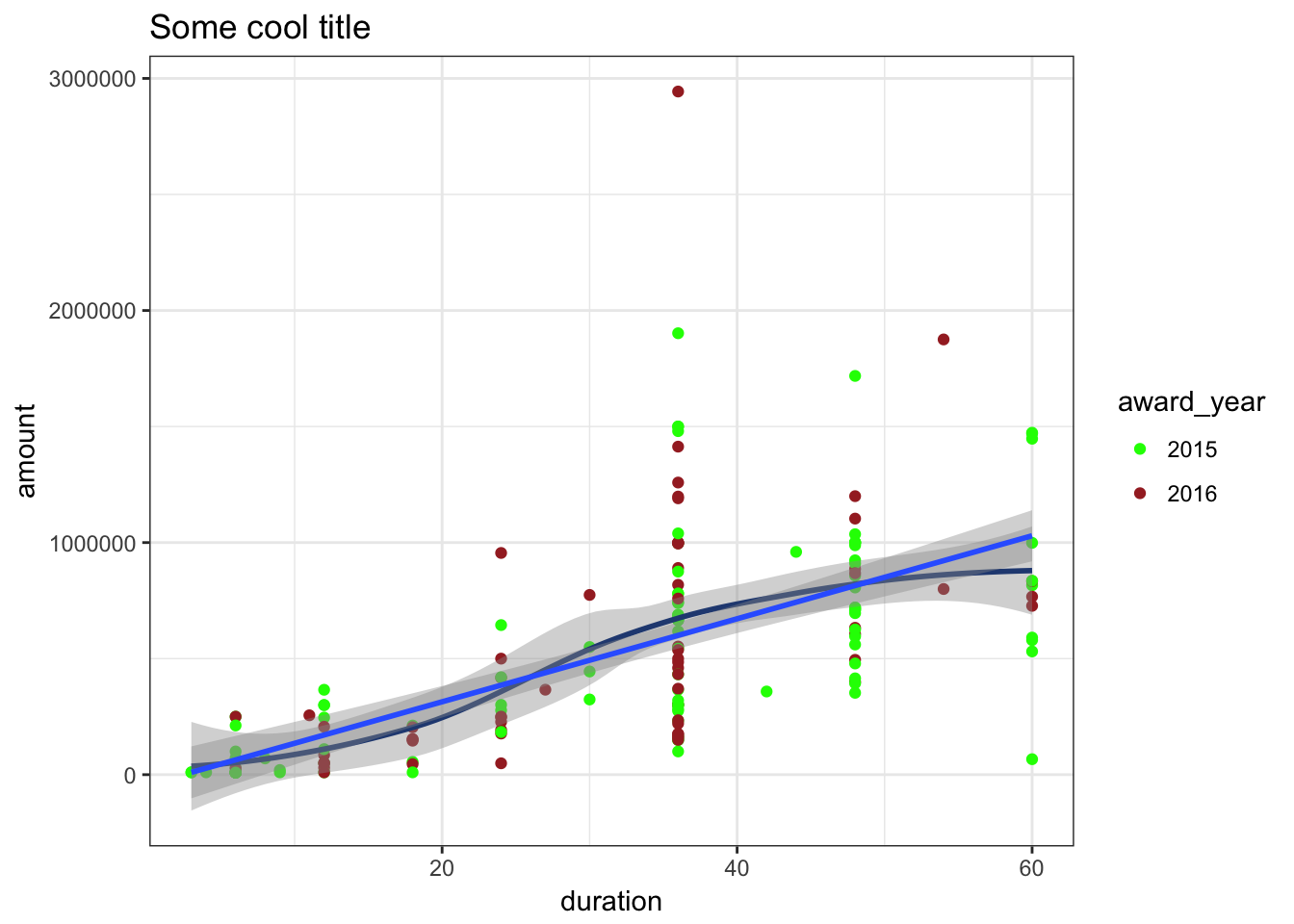… or saving the plot to your computer:

``````ggsave(p1, filename = "plot1.pdf")
ggsave(p2, filename = "plot2.png", width = 5, height = 7, units = "in")
ggsave(p3, filename = "plot3.pdf", width = 5, height = 7, units = "in")
# etc.``````

… or arranging multiple plots:

``````library(gridExtra)

grid.arrange(p1, p2, p3)``````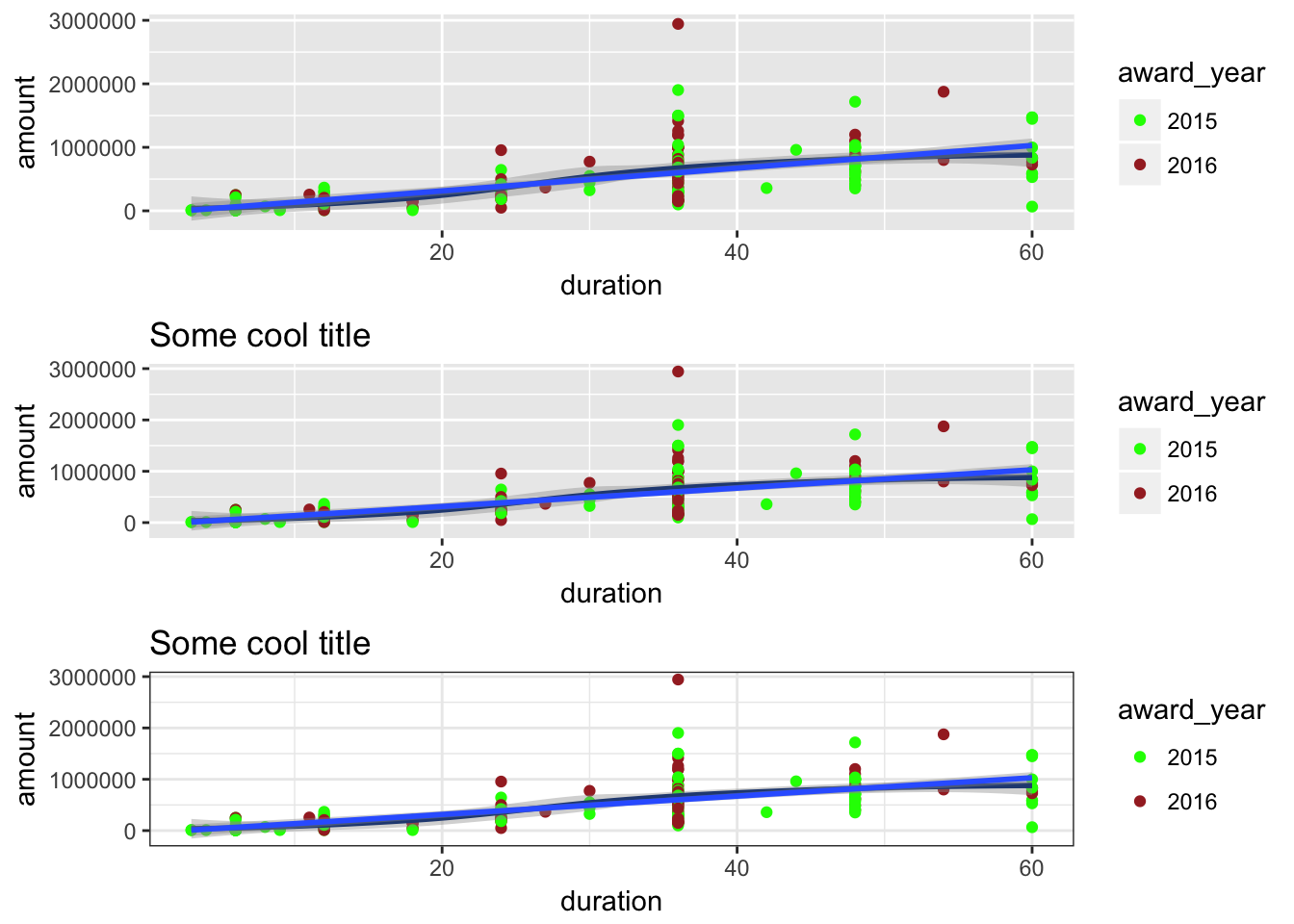``grid.arrange(p1, p2, p3, ncol = 1)````grid.arrange(p1, p2, p3, nrow = 3)``If you want to save one of these combined plots to an object, you need to use `arrangeGrob()` instead of `grid.arrange()`:

``p_lots_of_them <- arrangeGrob(p1, p2, p3, nrow = 3)``
``ggsave(p_lots_of_them, filename = "lots_o_plots.pdf")``

If you want to plot a saved `arrangeGrob` object, you have to use these two functions:

``````grid::grid.newpage()  # Clear out whatever plot is there already
grid::grid.draw(p_lots_of_them)  # Draw the combined plot``````### Fonts

It’s relatively easy to use custom fonts in `ggplot` plots, but there are some tricks. Read this post for more details and for instructions for how to do it on macOS and Windows.

### Colors

You can specify colors in R in a few different ways:

• R has hundreds of built-in named colors, listed here I typically don’t use these, except for the `greyXX` colors, since it’s easier to use something like `c("grey30", "grey60", "grey90")` to get a series of greyscale colors than looking up their hex codes.

• You can use hex codes (`#203864`, `#DC5B44`, etc.) or RGB values (`rgb(0, 146, 255)`)
• You can use special scales that draw on fancy algorithms and hand-picked color palettes, like Color Brewer and Viridis
``````library(viridis)
ggplot(cr_filtered, aes(x = duration, y = amount)) +
geom_point(aes(color = as.factor(program))) +
scale_color_viridis(discrete = TRUE)``````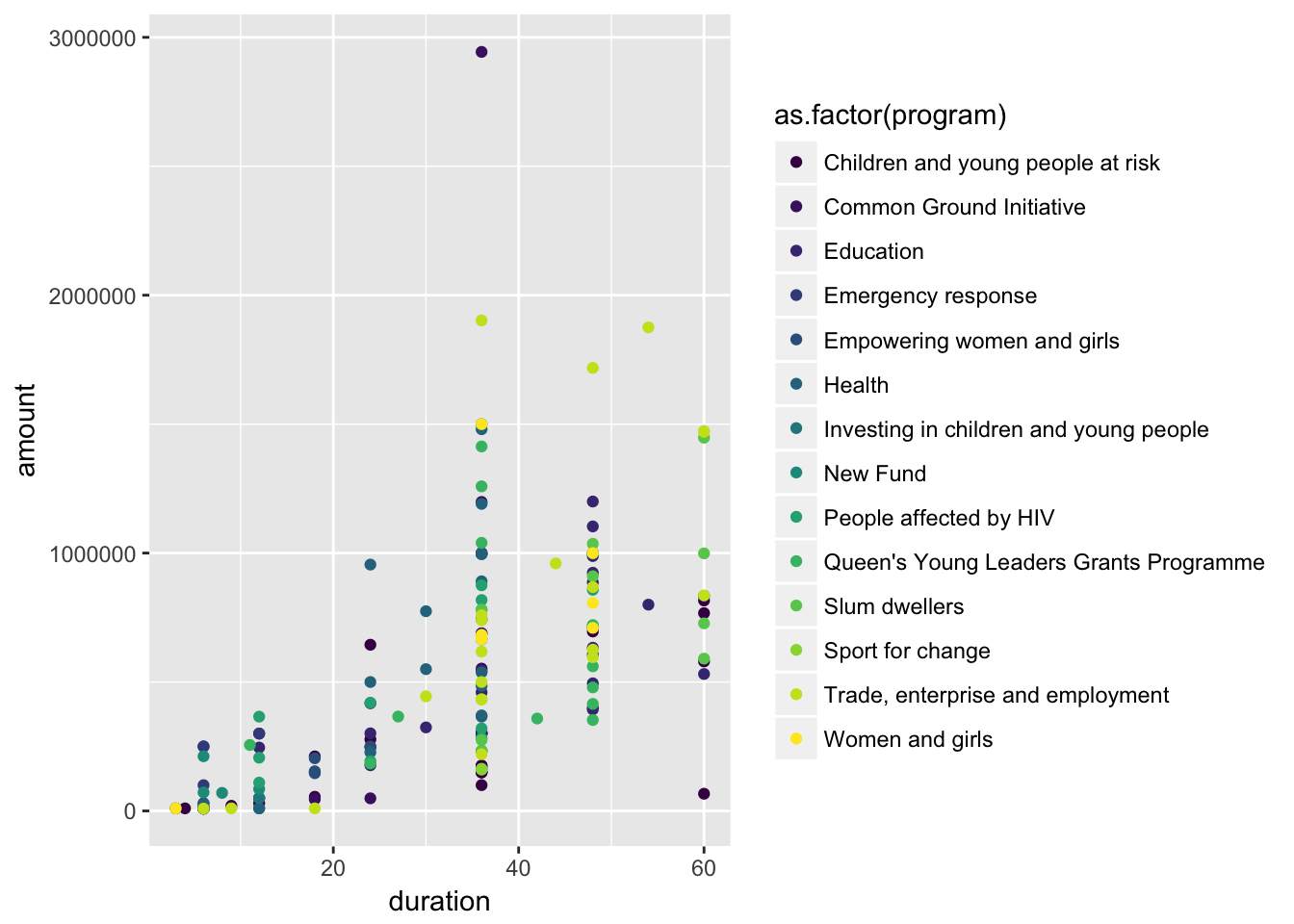``````ggplot(cr_filtered, aes(x = duration, y = amount)) +
geom_point(aes(color = as.factor(program))) +
scale_color_brewer(type = "qual")``````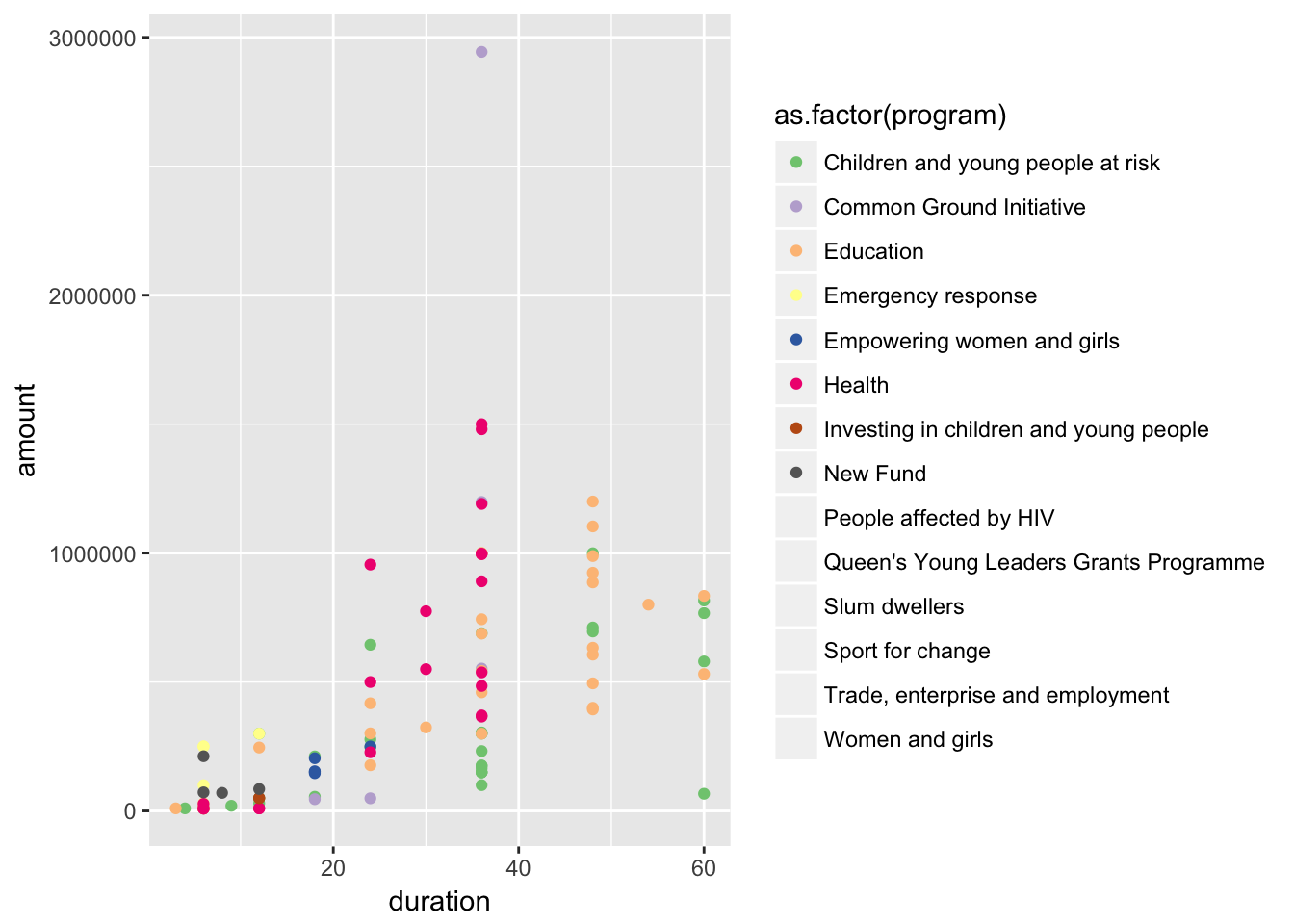``````ggplot(cr_filtered, aes(x = duration, y = amount)) +
geom_point(aes(color = as.factor(award_year))) +
scale_color_manual(values = c("#203864", "#DC5B44"))``````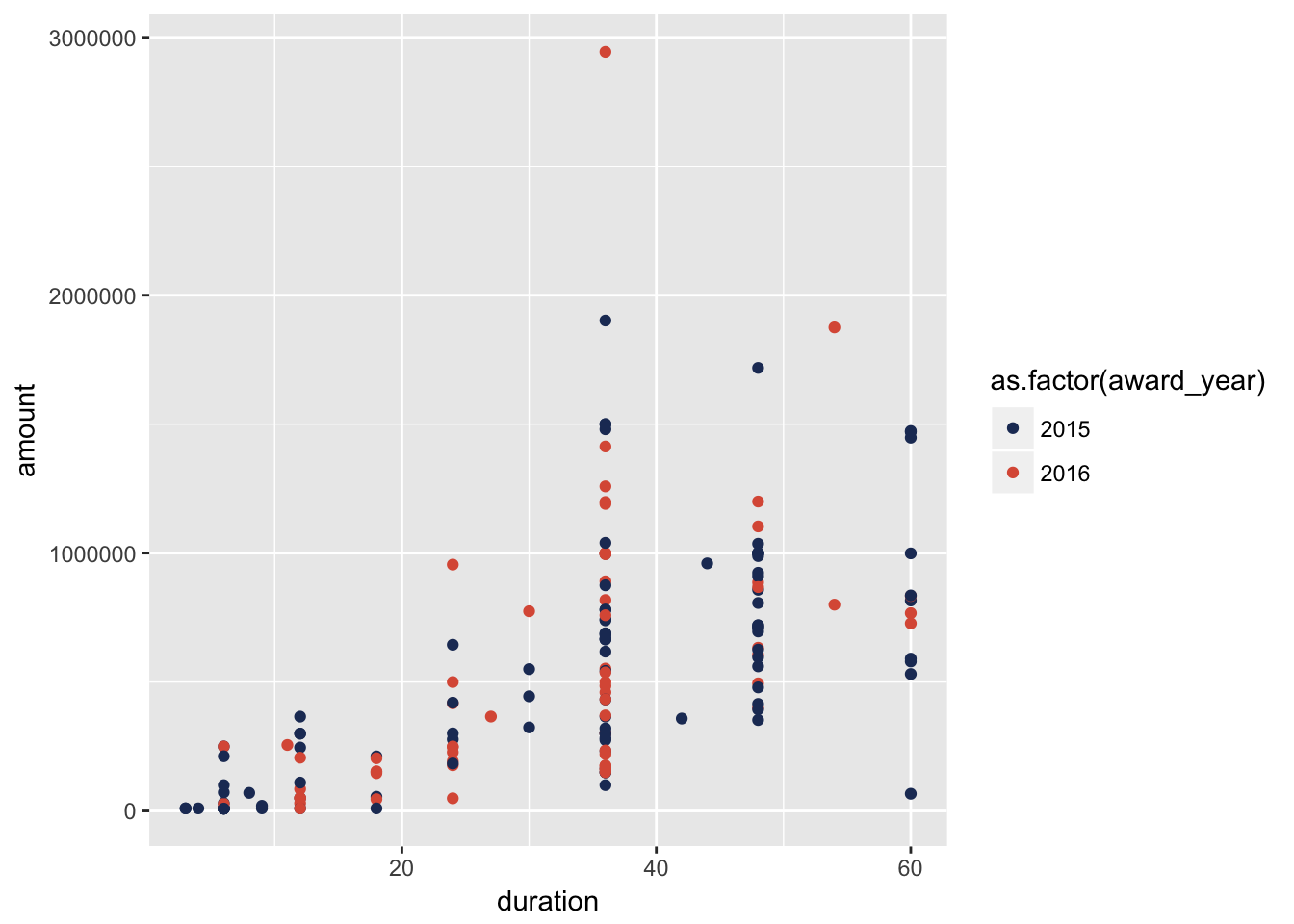### Theme options

You can make adjustments to plots with the `theme()` function, but there are a billion different options to play with. To simplify life, you can install a GUI interface for manipulating themes with the `ggThemeAssist` package. Once it’s installed, you can access it in RStudio with the “Addins” menu at the top of the window. You must have a ggplot object selected for it to work.

`ggThemeAssist` will generate a bunch of settings inside a `theme()` function. If you want to reuse those settings in other plots, you can assign them to a variable and then add that variable as a layer to other plots:

``````# This was all generated with ggThemeAssist
my_ugly_theme <- theme(axis.text.x = element_text(angle = 45),
panel.background = element_rect(fill = "darkgoldenrod1"),
plot.background = element_rect(fill = "antiquewhite"),
legend.background = element_rect(fill = "hotpink"),
legend.position = "bottom", legend.direction = "horizontal")

p1 + labs(x = "Months", y = "Amount", title = "Cool stuff") +
my_ugly_theme``````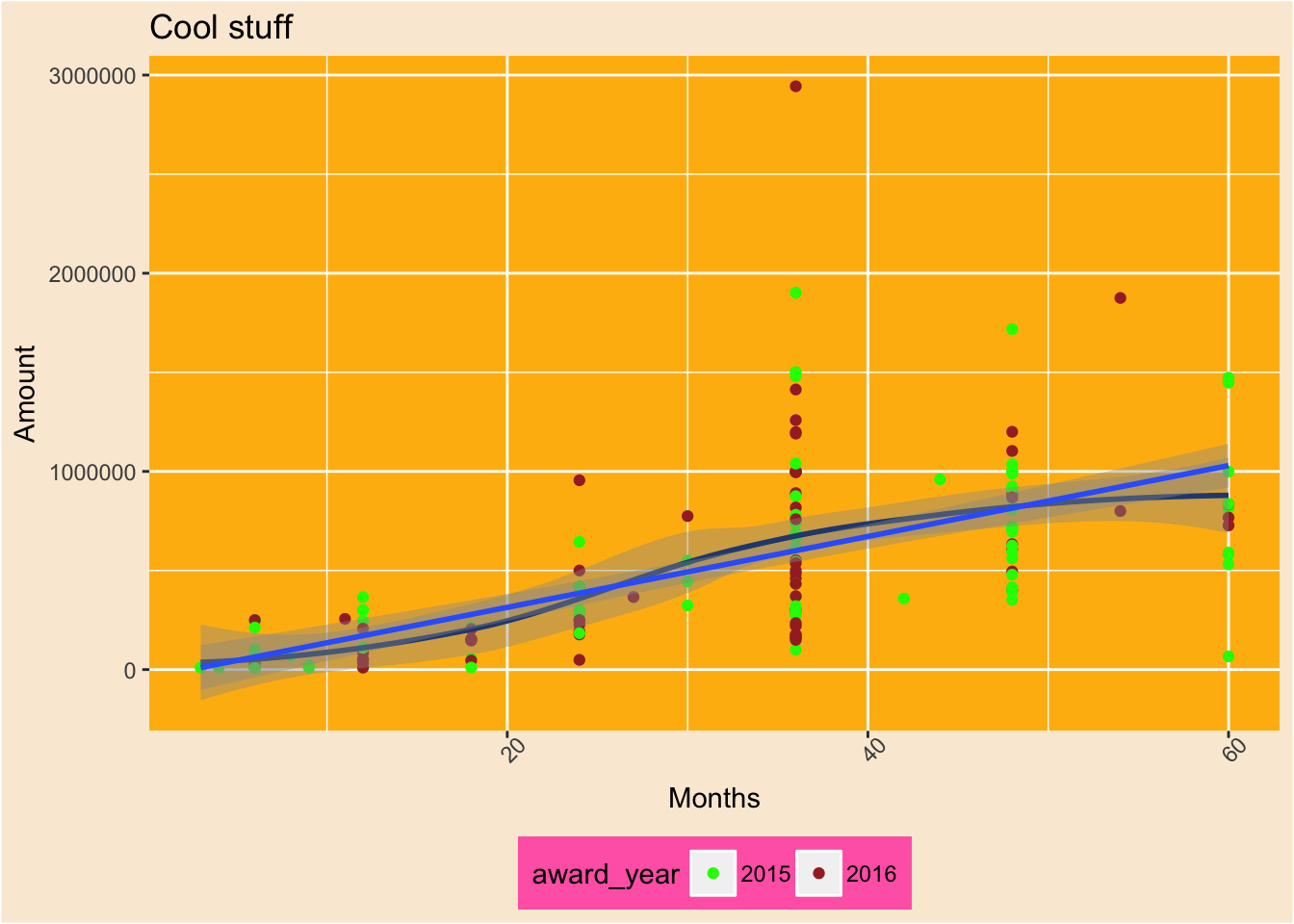## Feedback for today

Go to this form and answer these three questions (anonymously if you want):

1. What new thing did you learn today?
2. What was the most unclear thing about today?
3. What was the most exciting thing you learned today?